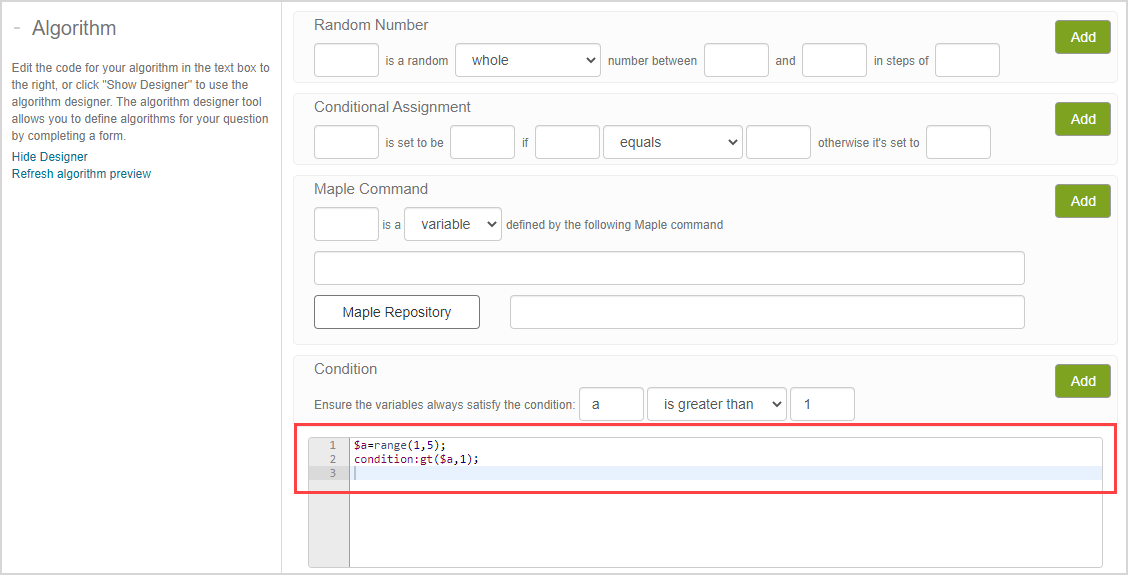Sometimes, inefficient custom algorithms can lead to system performance issues.

You can prevent these performance issues by optimizing your algorithm.

## Conditions

You can optimize conditions within your algorithm by:

• Using conditions sparingly
• Placing conditions as early in your algorithm as possible

NOTE: When a condition fails, the entire algorithm starts over causing a loop that continues until all conditions pass.

TIP: Be sure to eliminate unnecessary conditions whenever possible. Example — Consider the following algorithm:Copy this code
```1
2
`\$a=range(1,5);condition:gt(\$a,1);````

The same algorithm can be achieved without a condition:

Copy this code
```1
`\$a=range(2,5);````

TIP: Conditions can't always be eliminated from your algorithm (Example — a condition would be required if your algorithm is generating random numbers x and y with the requirement that |x^2*y/(x^4+y^2)| be less than 0.48). Conditions should be placed as early as possible within your algorithm when they can't be eliminated. Since the maple("...") function is often used to perform complex calculations, it should be placed after the last condition since this means that all of the algorithm's conditions have been met by the time the maple("...") function is applied.

## maple("...") function

Sending custom commands to the Maple™ engine allows you to create unique algorithms.

However, note that:

• Computations that take a long time to execute will affect the response time experienced by your students, and are capped at 20 seconds.
• Each individual call to the Maple™ engine using the maple("...") function introduces some overhead.

You can then optimize the usage of the maple("...") function within your algorithm by:

• Using the maple("...") function sparingly
• Returning the results of multiple commands (separated by commas) within one maple("...") function

TIP: You can obtain multiple results by placing multiple commands within one maple("...") function and extracting the results via the switch() command (check out the switch(n, a, b, c, ...) section of the View available functions for algorithmic questions help topic for how to use the switch() command). The switch() command means that numerous separate maple("...") calls don't have to be queued up and separate answers can be retrieved. Example — Consider this algorithm structure:

Copy this code
```1
2
3
4
`\$a=maple("command1,command2,command3;");\$var1=switch(0,\$a);\$var2=switch(1,\$a);\$var3=switch(2,\$a);````

This algorithm will output the results of command1 as \$var1, command2 as \$var2, and command3 as \$var3.

Example — Consider this algorithm that follows the multiple command structure:

Copy this code
```1
2
3
4
5
6
7
`\$seed=rint(10000);\$m=maple("randomize(\$seed):          poly:=randpoly(x,terms=4):          poly,MathML[ExportPresentation](poly),diff(poly,x);");\$poly=switch(0,\$m);\$polydisp=switch(1,\$m);\$deriv=switch(2,\$m);````